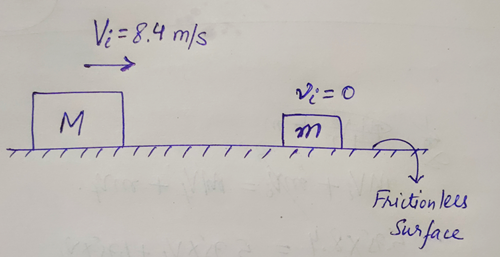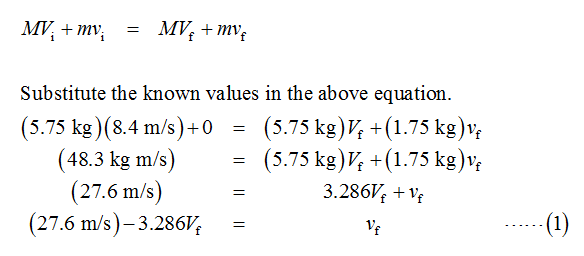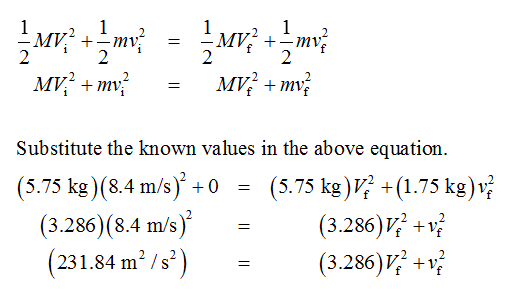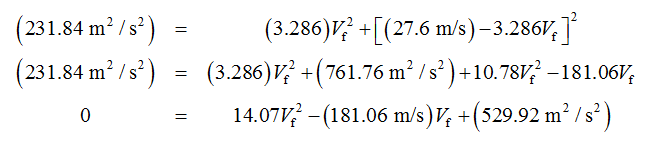# A block with mass M = 5.75 kg is sliding in the positive x-direction at Vi = 8.40 m/s on a frictionless surface when it collides elastically in one dimension with a stationary block with mass m = 1.75 kg.Determine the velocities, Vf and vf, of the objects after the collision.Vf= m/svf= m/s

Question
2 views

A block with mass M = 5.75 kg is sliding in the positive x-direction at Vi = 8.40 m/s on a frictionless surface when it collides elastically in one dimension with a stationary block with mass m = 1.75 kg.

Determine the velocities,

Vf

and

vf,

of the objects after the collision.

 Vf = m/s vf = m/s
check_circle

Step 1

Consider the diagram given below for the given system.Here, vi is zero because the mass m is at rest initially.

Since the collision is elastic, the linear momentum of the system is conserved.

Thus,Step 2

For the elastic collision total kinetic energy of the system before and after the collision is conserved.

Thus,Substitute the value of vf in the above equation from equation (1)....

### Want to see the full answer?

See Solution

#### Want to see this answer and more?

Solutions are written by subject experts who are available 24/7. Questions are typically answered within 1 hour.*

See Solution
*Response times may vary by subject and question.
Tagged in